Open in App
Not now

# Filtering a PySpark DataFrame using isin by exclusion

• Last Updated : 29 Jun, 2021

In this article, we will discuss how to filter the pyspark dataframe using isin by exclusion.

isin(): This is used to find the elements contains in a given dataframe, it takes the elements and gets the elements to match the data.

Syntax: isin([element1,element2,.,element n)

Creating Dataframe for demonstration:

## Python3

 `# importing module``import` `pyspark`` ` `# importing sparksession from pyspark.sql module``from` `pyspark.sql ``import` `SparkSession`` ` `# creating sparksession and giving an app name``spark ``=` `SparkSession.builder.appName(``'sparkdf'``).getOrCreate()`` ` `# list  of students  data  with null values``# we can define null values with none``data ``=` `[[``1``, ``"sravan"``, ``"vignan"``],``        ``[``2``, ``"ramya"``, ``"vvit"``],``        ``[``3``, ``"rohith"``, ``"klu"``],``        ``[``4``, ``"sridevi"``, ``"vignan"``],``        ``[``5``, ``"gnanesh"``, ``"iit"``]]`` ` `# specify column names``columns ``=` `[``'ID'``, ``'NAME'``, ``'college'``]`` ` `# creating a dataframe from the lists of data``dataframe ``=` `spark.createDataFrame(data, columns)``dataframe.show()`

Output: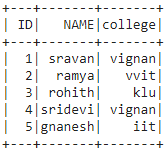Method 1: Using filter()

filter(): This clause is used to check the condition and give the results, Both are similar

Syntax: dataframe.filter(condition)

Example 1: Get the particular ID’s with filter() clause

## Python3

 `# get the ID : 1,2,3 from dataframe``dataframe.``filter``((dataframe.``ID``).isin([``1``,``2``,``3``])).show()`

Output: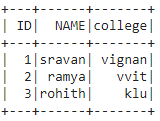Example 2: Get names from dataframe columns.

## Python3

 `# get name as sravan``dataframe.``filter``((dataframe.NAME).isin([``'sravan'``])).show()`

Output: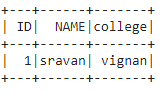Method 2: Using Where()

where(): This clause is used to check the condition and give the results

Syntax: dataframe.where(condition)

Example 1: Get the particular colleges with where() clause.

## Python3

 `# get college as vignan``dataframe.where((dataframe.college).isin([``'vignan'``])).show()`

Output: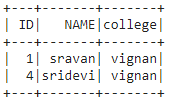Example 2: Get ID except 5 from dataframe.

## Python3

 `# get ID except 1``dataframe.where(~(dataframe.``ID``).isin([``1``])).show()`

Output: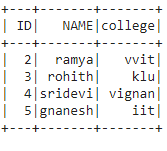My Personal Notes arrow_drop_up